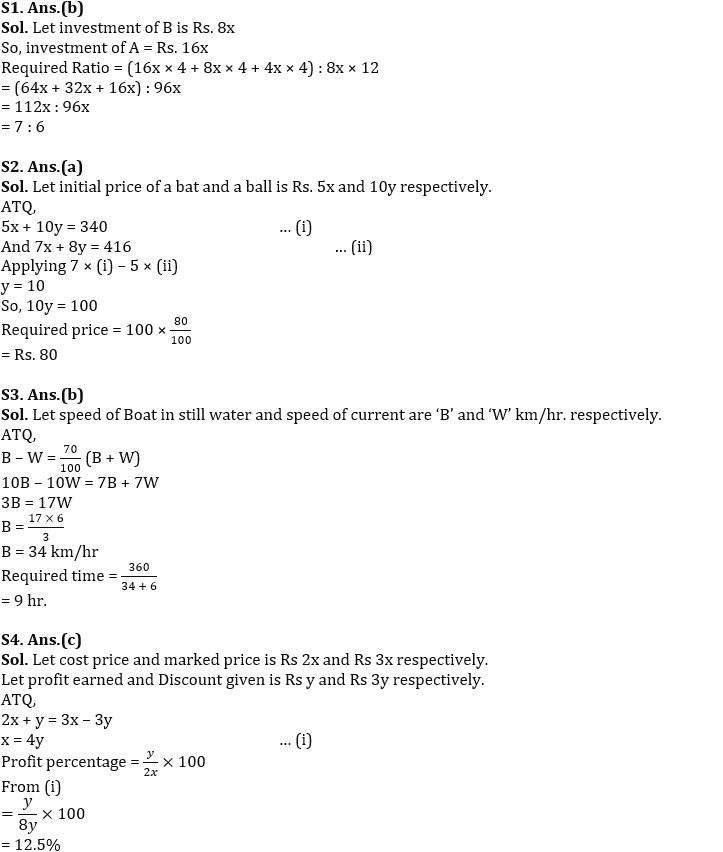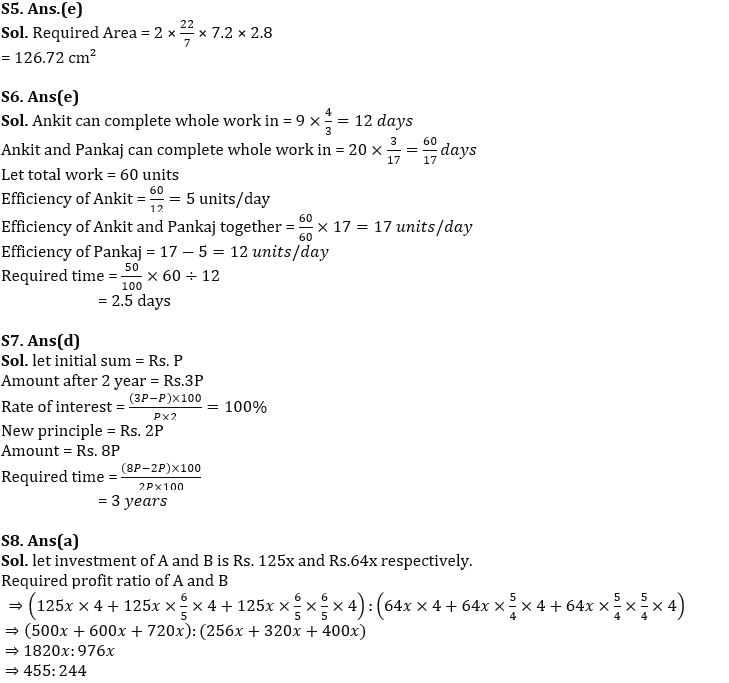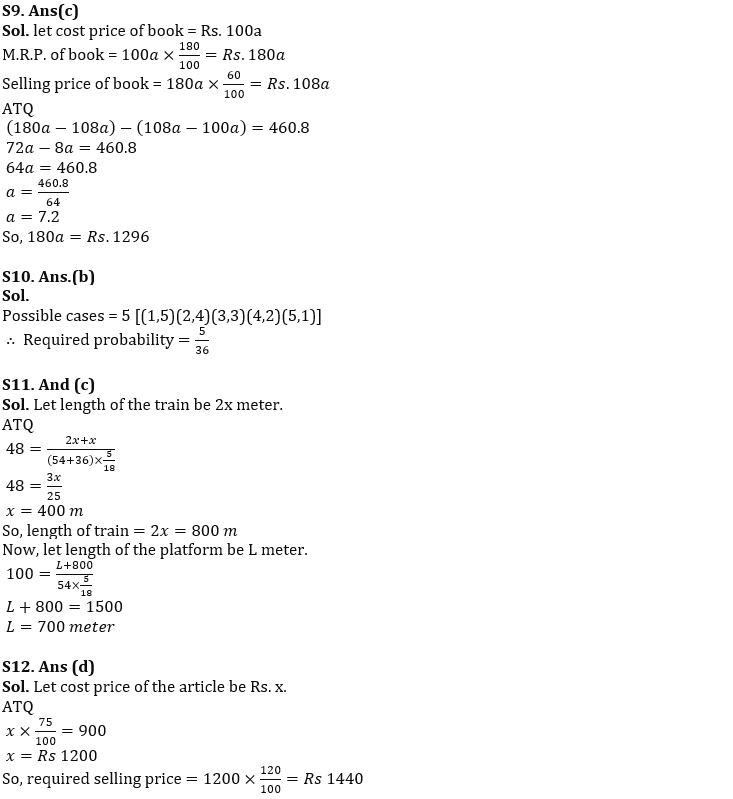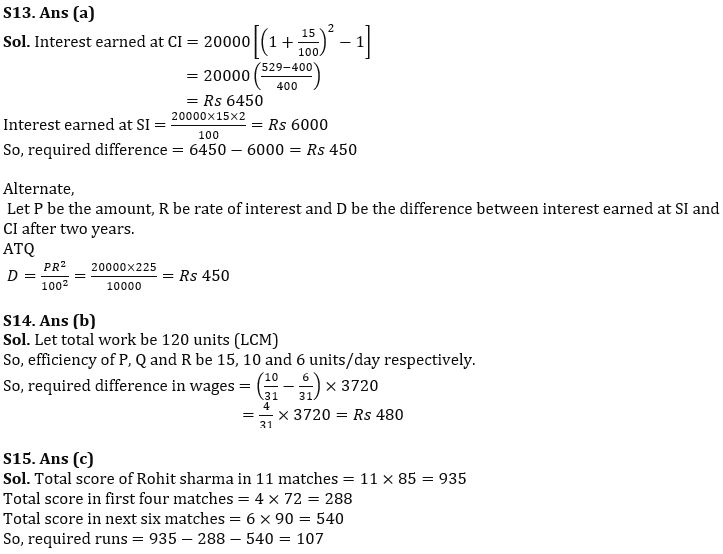Latest Banking jobs   »

# Quantitative Aptitude Quiz For FCI Phase I 2022- 23rd September

Q1. A invest twice the sum invested by B and withdraws half of sum after 4 months and again withdraws half of the remaining sum after 4 months. Find Ratio of profit share of A to B at the end of a year?
(a) 3 : 4
(b) 7 : 6
(c) 4 : 3
(d) 6 : 7
(e) None of these

Q2. Total Price of a bat and a ball is Rs. 340, when price of bat is increased by 40% and price of ball is decreased by 20% then total price become Rs. 416. Find decreased price of a ball?
(a) Rs. 80
(b) Rs. 90
(c) Rs. 72
(d) Rs. 100
(e) Rs. 96

Q3. Speed of a boat in upstream is 70% of speed of boat in downstream. If speed of current is 6 km/hr. Find time taken by boat in downstream to cover 360 km?
(a) 7 hr
(b) 9 hr
(c) 8 hr
(d) 11 hr
(e) 10 hr

Q4. Ratio of cost price to marked price is 2 : 3 and Ratio of Discount given to profit earned is 3 : 1, find profit percentage.
(a) 37.5%
(b) 25%
(c) 12.5%
(d) 16.67%
(e) 50%

Q5. What is the curved surface area of a cylinder whose height and radius is 2.8 cm and 7.2 cm respectively?
(a) 63.36 cm²
(b) 158.4 cm²
(c) 168.96 cm²
(d) None of these
(e) 126.72 cm²’

Q6. Ankit can do 3/4 th of work in 9 days whereas Pankaj and Ankit working together can do 5⅔ times of same work in 20 days. Find in how many days Pankaj can complete 50% of the same work?
(a) 2 days
(b) 3 days
(c) 4 days
(d) 3.5 days
(e) 2.5 days

Q7. A sum become 3 times in 2 years at rate of simple interest. If twice of that sum is invested, then find in how many years the sum will become 8 times of initial sum at same rate of simple interest?
(a) 2 years
(b) 4 years
(c) 7 years
(d) 3 years
(e) Can’t be determined.

Q8. A and B invested in a partnership in ratio of 125:64 and after every 4-month, A and B increases their investment by 20% and 25% respectively. Find ratio of their profit at the end of the year?
(a) 455:244
(b) 244:279
(c) 93:97
(d) 61:81
(e) None of these.

Q9. A book is marked 80% above cost price and sold at 40% discount, if difference between discount given and profit earned is Rs.460.8, then find M.R.P. of book?
(a) Rs.720
(b) Rs.1200
(c) Rs.1296
(d) Rs. 777.6
(e) Rs.1660

Q10. If two dices are rolled simultaneously, then find the probability of getting a sum equal to 6.
(a) 4/9
(b) 5/36
(c) 5/9
(d) 13/36
(e) None of these.

Q11. A train travelling at 54 kmph crosses another train, having half of its length and travelling in opposite direction at 36 kmph in 48 seconds. It also passed a railway platform in 100 seconds. Find the length of the platform.
(a) 400 m
(b) 600 m
(c) 700 m
(d) 900 m
(e) 500 m

Q12. By selling an article at Rs 900, a shopkeeper gets a loss of 25%. Find, to make a profit of 20% he should sell the article at how much price?
(a) Rs 1200
(b) Rs 1320
(c) Rs 1180
(d) Rs 1440
(e) Rs 1560

Q13. Find the difference between interest earned on amount of Rs. 20000 at CI and SI at same rate of interest of 15% per annum and for same time of 2 years.
(a) Rs 450
(b) Rs 540
(c) Rs 620
(d) Rs 480
(e) Rs 520

Q14. P, Q and R can do a piece of work in 8 days, 12 days and 20 days respectively. If total wage earned by them is Rs 3720, then find wage of Q is how much more/less than R.
(a) Rs 520
(b) Rs 480
(c) Rs 660
(d) Rs 620
(e) Rs 450

Q15. In a series of 11 matches of Cricket, average runs of Rohit sharma is 85. In first 4 matches his average runs was 72 and in next 6 matches his average runs was 90. How many runs scored by Rohit sharma in last match?
(a) 115
(b) 120
(c) 107
(d) 97
(e) 112

Solutions#### Congratulations!Download Hindu Review of October 2021: Free PDF